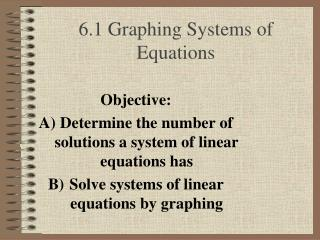DownloadDownload Presentation6.1 Graphing Systems of Equations

6.1 Graphing Systems of Equations

Download Presentation6.1 Graphing Systems of Equations

- - - - - - - - - - - - - - - - - - - - - - - - - - - E N D - - - - - - - - - - - - - - - - - - - - - - - - - - -
Presentation Transcript

1. 6.1 Graphing Systems of Equations Objective: Determine the number of solutions a system of linear equations has Solve systems of linear equations by graphing

2. How do we graph a Linear Equation Linear Equation- y=mx + b 1) Find the y-intercept 2) Use the slope to find your next point 3) Draw a line through the two points

3. What is a system of equations? A "system" of equations is a set of 2 or more equations that we solve as one.

4. Vocabulary • Consistent-a system that has at least one solution. The graphs intersect at one point or are the same line. • Inconsistent-a system that has no solution

5. Vocabulary • Independent-a consistent system that has exactly one solution • Dependent-a consistent system that has an infinite number of solutions

6. Possible Solutions

7. You Try Page 336 #1-6

8. Finding a Solution by Graphing • Graph the equations carefully on the same coordinate plane • Find their point of intersection a) This point is the solution of the system

9. Example 1 Graph each system and determine the number of solutions that it has. If it has one solution, name it. y = -3x +10 y = x –2

10. Example 2 Graph the system and determine the solution to the system. 2x - y = -1 4x – 2y = 6

11. Example 3 y = -2x – 3 6x + 3y = -9

12. You Try Page 336 # 7 and 8

13. Write and Solve a System of Equations Naresh rode 20 miles last week and plans to ride 35 miles per week. Diego rode 50 miles last week and plans to ride 25 miles per week. Predict the week in which Naresh and Diego will have ridden the same number of miles.

14. Write and Solve a System of Equations Joe and Josh each want to buy a video game. Joe has \$14 and saves \$10 a week. Josh has \$26 and saves \$7 a week. In how many weeks will they have the same amount?

15. You Try Page 336 #9

16. Journal Describe the advantages and disadvantages to solving systems of equations by graphing

17. Class work Page 336 #11- 25 odd